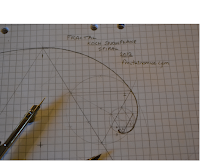## Posts

Showing posts from April, 2012

### Fractal Koch Snowflake SpiralThe Koch Snowflake Spiral On route to understanding if   fractals have a 'wavy' like nature , I finally put pencil to paper and drew what I've been thinking. I have been pondering on what effect a 'mutation' or change to one triangle - a dot at the apex of the triangle as shown below - would have or show on the  iterating  Koch Snowflake. I envisaged that it would spiral to infinity as shown below. By tracing a smooth curve through the (red) 'dots' series of iterated mutant triangles, I would develop - what looks like - a kind of logarithmic spiral , or a wave or pulse if this spiral curve was viewed in the front elevation view as opposed to the plan elevation (above). Method As a circles radius (what the compass is set to) scribes  6 times around the circles circumference , the three corners of the 1st equilateral triangle (iteration 1) can be produced, and the apex of the 2nd triangle (iteration 2) can also be marked. With the compass st

### Fractal: MultiplierDevelopment, and growth of the fractal demonstrates the ( money and Keynesian) Multiplier. The (Keynesian) Multiplier shows how an initial injection of expenditure into an economic system goes on to create more income. This is because added expenditure – in itself – sets off additional rounds of expenditure with each and every hand or round this income passes. This principle of 'multiplying' the initial injection can be demonstrated by use of the fractal: In the diagram below: income is represented by the area of each and every triangle, and the rounds of spending by every iteration of the rule.     The initial injection (iteration 1) is represented by the first triangle – which has an arbitrary area of 1. The next round adds 3 extra triangles, increasing the total area of the snowflake. This (principle) process continues until the changes in area of the triangle  – after  each iteration  –  changes the total area of the snowflake no more. In the diagram above t